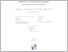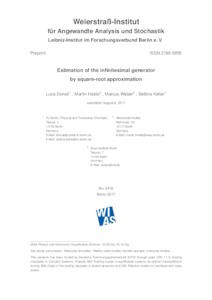Repository: Freie Universität Berlin, Math Department

# Estimation of the infinitesimal generator by square-root approximation

Donati, L. and Heida, M. and Weber, M. and Keller, B. (2018) Estimation of the infinitesimal generator by square-root approximation. Journal of Physics: Condensed Matter, 30 (42). p. 425201. ISSN 0953-8984, ESSN: 1361-648XPreview

1MB

## Abstract

For the analysis of molecular processes, the estimation of time-scales, i.e., transition rates, is very important. Estimating the transition rates between molecular conformations is -- from a mathematical point of view -- an invariant subspace projection problem. A certain infinitesimal generator acting on function space is projected to a low-dimensional rate matrix. This projection can be performed in two steps. First, the infinitesimal generator is discretized, then the invariant subspace is approxi-mated and used for the subspace projection. In our approach, the discretization will be based on a Voronoi tessellation of the conformational space. We will show that the discretized infinitesimal generator can simply be approximated by the geometric average of the Boltzmann weights of the Voronoi cells. Thus, there is a direct correla-tion between the potential energy surface of molecular structures and the transition rates of conformational changes. We present results for a 2d-diffusion process and Alanine dipeptide.

Item Type: Article SFB 1114 Preprint 08/2017 im WIAS (2416) Mathematical and Computer Sciences > Mathematics > Applied Mathematics 2167 Silvia Hoemke 19 Dec 2017 13:30 01 Nov 2018 13:55

Repository Staff Only: item control page